# Mathematics needs logic to be formulated

## Logic¶

The (propositional) logic is of fundamental importance for all sub-areas of mathematics.

With the help of connecting words such as “and”, “or”, “exactly if” etc., several (partial) statements can be linked to form a compound statement. The following statement functions can be used in logic two (or more) statements too one form a new statement.

The conjunction

If you combine two statements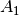and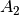the word “and” creates the conjunction of the statementsand, symbolically with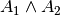designated.

A conjunction of two statements is therefore only true if both (partial) statements are true.

Examples:

If you combine two statementsandthe word “or” creates the adjunction of the statementsand, symbolically with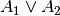designated.

The adjunction is therefore true if one of the two statements is true (or both are true).

Examples:

• The adjunct of the true statement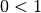and the wrong statement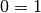is the true statement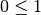.
• The true statement: "Either the earth is a cube or the sun is a star" is an adjunct of the false statement: "The earth is a cube" and the true statement: "The sun is a star".

The implication

If you combine two statementsandthe word “then” creates the implication of the statementsand, symbolically with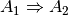designated.

The implication is true if both statementsand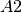are true or if the first statementis wrong.  Formally, one obtains an identical truth table if one uses the implication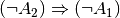forms.  

Examples:

Equivalence of two statements

If you combine two statementsandthe word combination “then, and only then” creates the equivalence of the statementsand, symbolically with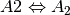designated.

The equivalence of two partial statements is only true if either both partial statements are true or both are false. 

Examples:

• The true statement "In a right-angled triangle the rate of height applies" equivalently linked to the false statement "In a right-angled triangle all sides are of the same length" results in the false statement "In a right triangle all sides are the same length if and only if the rate of height applies" .
• The equivalence combination of the false statement “The kilogram is a unit of length” with the true statement “A thousand meters equals one kilometer” is the false statement “The kilogram is a unit of length if and only then, if a thousand meters equals a kilometer”.

Contravalence of two statements

If you combine two statementsandthe word “either or” in the exclusive sense creates the contravalence of the statementsand, with with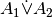designated.

The contravalence of two partial statements is only true if exactly one of the two (partial) statements is true. Thus, as its name suggests, it is formally identical with the negation of equivalence.

Example:

• If you combine the true statement “The train is going to Munich” with the false statement “The train is going to Frankfurt”, the result is the true statement “The train is either going to Munich or to Frankfurt”.

Rules for the combination of statements

The following equivalences are defined between the statements and their links, some of which have a formal similarity to the rules for calculating with numbers: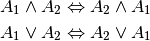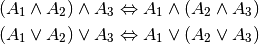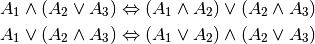In addition, there are the following rules, which are sometimes useful for evidence proceedings and in computer science: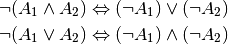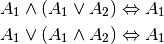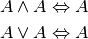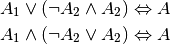In doing so, the link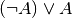also called "tautology"; it is always true. 

### Variables, terms and proposition forms¶

A variable is a symbol for any element from a given basic set. In addition, there are no special rules or laws for calculating with variables.

A term is a designation on the one hand for a single mathematical object (for example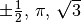), on the other hand also for a string of several constants, variables, brackets and arithmetic operators (for example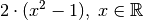).  However, terms do not contain a relational sign, so they are neither true nor false.

In addition to (at least) one variable and (at least) one term, a statement form always contains a relation symbol - for example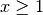or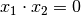. However, in order to be able to assign a truth value to a statement form, all occurring variables must first be replaced by concrete elements from the basic set. Just like statements, several types of statements can be combined to create new types of statements through logical links.

The dependence of a statement form on one or more variables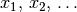will be in the form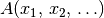expressed. Statements can be divided into three types:

Forms of assertion are treated as equations and inequalities, especially in algebra.

'For all' and 'There is'

In addition to inserting specific objects for the occurring variables, forms of statements can also be made into statements in a second way: quantification.

While an existence statement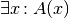is true if the underlying statement form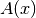even just for a specific one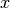is fulfilled, a universal statement can be made in the opposite case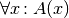already through the proof of existence of a single "counterexample"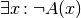be refuted as false.  

### Direct and indirect evidence¶

The formal rules of logic can also be used in order to be able to draw conclusions about new laws using forms of statements that have already been proven to be true. Theorems obtained in this way (also called “theorems” or “propositions” for short) represent the basic structure of mathematical theory.

In addition to already known theorems, so-called definitions are also used in order to be able to prove new theorems. When defining a term, it is clearly defined by defining essential, common features and is differentiated from other terms. Definitions are neither true nor false; rather, they serve as abbreviations for awkward formulations. The symbol is used as a definition symbol for mathematical terms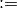, an abbreviation for "is equal by definition".

For the actual "proofs", the following conclusive rules of logic are possible, among others:

• Conclusion from a negation:
The propositional expression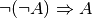is generally valid. A proposition can thus be proven by refuting the negation of the proposition.

In the case of direct proofs, based on valid prerequisites and using admissible inference rules, after a finite number of steps, the assertion is inferred directly. In the case of indirect proofs, on the other hand, the negation of the assertion is added to the prerequisites.

The complete induction

Complete induction is a widely used technique for directly proving a statement. The logical conclusion is based on three steps:

1. With the "induction start" it is shown that a statement formfor a (freely selectable) value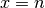is valid.
2. The "induction assumption" consists in the fact that the statement formfor a particular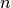is valid.
3. With the “induction conclusion”, a “proof in the proof”, it is shown that from the validity of the statement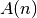also the validity of the statement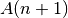follows, in shorthand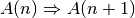.

Example:

Remarks: